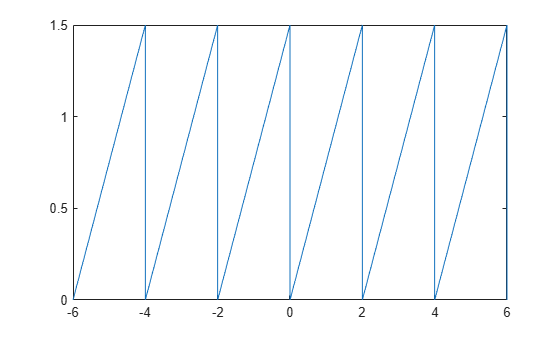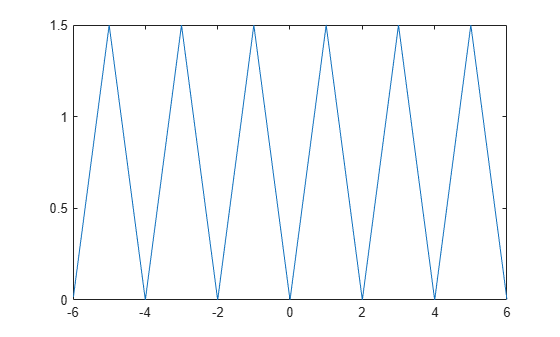# mod

Symbolic modulus after division

Starting in R2020b, `mod` no longer finds the modulus for each coefficient of a symbolic polynomial. For more information, see Compatibility Considerations.

## Syntax

``m = mod(a,b)``

## Description

example

````m = mod(a,b)` finds the modulus after division. To find the remainder, use `rem`.If `a` is a polynomial expression, then `mod(a,b)` returns the unevaluated modulus of the polynomial.```

## Examples

collapse all

Find the modulus after division when both the dividend and divisor are integers.

Find the modulus after division for these numbers.

`m = [mod(sym(27),4), mod(sym(27),-4), mod(sym(-27),4), mod(sym(-27),-4)]`
`m = $\left(\begin{array}{cccc}3& -1& 1& -3\end{array}\right)$`

Find the modulus after division when the dividend is a rational number, and the divisor is an integer.

Find the modulus after division for these numbers.

`m = [mod(sym(22/3),5), mod(sym(1/2),7), mod(sym(27/6),-11)]`
```m =  $\left(\begin{array}{ccc}\frac{7}{3}& \frac{1}{2}& -\frac{13}{2}\end{array}\right)$```

Find the modulus after division when the dividend is a polynomial expression, and the divisor is an integer. If the dividend is a polynomial expression, then `mod` returns a symbolic expression without evaluating the modulus.

Find the modulus after division by $10$ for the polynomial ${x}^{3}-2x+999$.

```syms x a = x^3 - 2*x + 999; mUneval = mod(a,10)```
`mUneval = `

To evaluate the modulus for each polynomial coefficient, first extract the coefficients of each term using `coeffs`.

`[c,t] = coeffs(a)`
`c = $\left(\begin{array}{ccc}1& -2& 999\end{array}\right)$`
`t = $\left(\begin{array}{ccc}{x}^{3}& x& 1\end{array}\right)$`

Next, find the modulus of each coefficient in `c` divided by `10`. Reconstruct a new polynomial using the evaluated coefficients.

```cMod10 = mod(c,10); mEval = sum(cMod10.*t)```
`mEval = ${x}^{3}+8 x+9$`

For vectors and matrices, `mod` finds the modulus after division element-wise. When both arguments are nonscalar, they must have the same size. If one argument is a scalar, the `mod` function expands the scalar input into an array of the same size as the other input.

Find the modulus after division for the elements of two matrices.

```A = sym([27,28; 29,30]); B = sym([2,3; 4,5]); M = mod(A,B)```
```M =  $\left(\begin{array}{cc}1& 1\\ 1& 0\end{array}\right)$```

Find the modulus after division for the elements of matrix `A` and the value `9`. Here, `mod` expands `9` into the `2`-by-`2` matrix with all elements equal to `9`.

`M = mod(A,9)`
```M =  $\left(\begin{array}{cc}0& 1\\ 2& 3\end{array}\right)$```

Create two periodic functions that represents sawtooth waves.

Define the sawtooth wave with period `T = 2` and amplitude `A = 1.5`. Create a symbolic function `y(x)`. Use `mod` functions to define the sawtooth wave for each period. The sawtooth wave increases linearly for a full period, and it drops back to zero at the start of another period.

```T = 2; A = 1.5; syms y(x); y(x) = A*mod(x,T)/T;```

Plot this sawtooth wave for the interval `[-6 6]`.

`fplot(y,[-6 6])`Next, create another sawtooth wave that is symmetrical within a single period. Use `piecewise` to define the sawtooth wave that is increasing linearly for the first half of a period, and then decreasing linearly for the second half of a period.

```y(x) = piecewise(0 < mod(x,T) <= (T/2), 2*A*mod(x,T)/T,... (T/2) < mod(x,T) <= T, 2*A - 2*A*mod(x,T)/T);```

Plot this sawtooth wave for the interval `[-6 6]`.

`fplot(y,[-6 6])`## Input Arguments

collapse all

Dividend (numerator), specified as a number, symbolic number, variable, polynomial expression, or a vector or matrix of numbers, symbolic numbers, variables, or polynomial expressions. Inputs `a` and `b` must be the same size unless one is a scalar. The function expands a scalar input into an array of the same size as the other input.

Divisor (denominator), specified as a number, symbolic number, or a vector or matrix of numbers or symbolic numbers. Inputs `a` and `b` must be the same size unless one is a scalar. The function expands a scalar input into an array of the same size as the other input.

collapse all

### Modulus

The modulus of a and b is

`$\mathrm{mod}\left(a,b\right)=a-b\text{\hspace{0.17em}}·\text{\hspace{0.17em}}\text{floor}\left(\frac{a}{b}\right),$`

where `floor` rounds (a / b) toward negative infinity. For example, the modulus of –8 and –3 is –2, but the modulus of –8 and 3 is 1.

If b = 0, then mod(a, b) = mod(a, 0) = 0.

## Tips

• Calling `mod` for numbers that are not symbolic objects invokes the MATLAB® `mod` function.

## Version History

Introduced before R2006a

expand all